# How to add metadata to a DataFrame or Series with Pandas in Python?

Metadata, also known as data about the data. Metadata can give us data description, summary, storage in memory, and datatype of that particular data. We are going to display and create metadata.

Scenario:

• We can get metadata simply by using info() command
• We can add metadata to the existing data and can view the metadata of the created data.

Steps:

• Create a data frame

Here, we are going to create a data frame, and we can view and create metadata on the created data frame

• dataframe_name.info() – It will return the data types null values and memory usage in tabular format
• dataframe_name.columns() – It will return an array which includes all the column names in the data frame
• dataframe_name.describe() – It will give the descriptive statistics of the given numeric data frame column like mean, median, standard deviation etc.

We can create the metadata for the particular data frame using dataframe.scale() and dataframe.offset() methods. They are used to represent the metadata.

Syntax:

dataframe_name.scale=value

dataframe_name.offset=value

Below are some examples which depict how to add metadata to a DataFrame or Series:

Example 1

Initially create and display a dataframe.

## Python3

 `# import required modules` `import` `pandas as pd`   `# initialise data of lists using dictionary` `data ``=` `{``'Name'``: [``'Sravan'``, ``'Deepak'``, ``'Radha'``, ``'Vani'``],` `        ``'College'``: [``'vignan'``, ``'vignan Lara'``, ``'vignan'``, ``'vignan'``],` `        ``'Department'``: [``'CSE'``, ``'IT'``, ``'IT'``, ``'CSE'``],` `        ``'Profession'``: [``'Student'``, ``'Assistant Professor'``,` `                       ``'Programmer & ass. Proff'``,` `                       ``'Programmer & Scholar'``],` `        ``'Age'``: [``22``, ``32``, ``45``, ``37``]` `        ``}`   `# create dataframe` `df ``=` `pd.DataFrame(data)`   `# print dataframe` `df`

Output: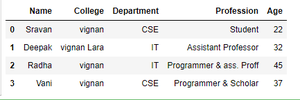Then check dataframe attributes and description.

## Python3

 `# data information` `df.info()`   `# data columns description` `df.columns`   `# describing columns` `df.describe()`

Output: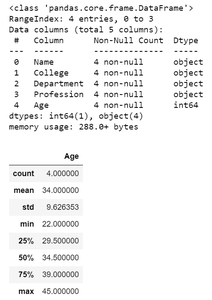Initialize offset and scale of the dataframe.

## Python3

 `# initializing scale and offset` `# for creating meta data` `df.scale ``=` `0.1` `df.offset ``=` `15`   `# display scale and offset` `print``(``'Scale:'``, df.scale)` `print``(``'Offset:'``, df.offset)`

Output: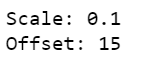We are storing data in hdf5 file format, and then we will display the dataframe along with its stored metadata.

## Python3

 `# store in hdf5 file format` `storedata ``=` `pd.HDFStore(``'college_data.hdf5'``)`   `# data` `storedata.put(``'data_01'``, df)`   `# including metadata` `metadata ``=` `{``'scale'``: ``0.1``, ``'offset'``: ``15``}`   `# getting attributes` `storedata.get_storer(``'data_01'``).attrs.metadata ``=` `metadata`   `# closing the storedata` `storedata.close()`   `# getting data` `with pd.HDFStore(``'college_data.hdf5'``) as storedata:` `    ``data ``=` `storedata[``'data_01'``]` `    ``metadata ``=` `storedata.get_storer(``'data_01'``).attrs.metadata`   `# display data` `print``(``'\nDataframe:\n'``, data)`   `# display stored data` `print``(``'\nStored Data:\n'``, storedata)`   `# display metadata` `print``(``'\nMetadata:\n'``, metadata)`

Output: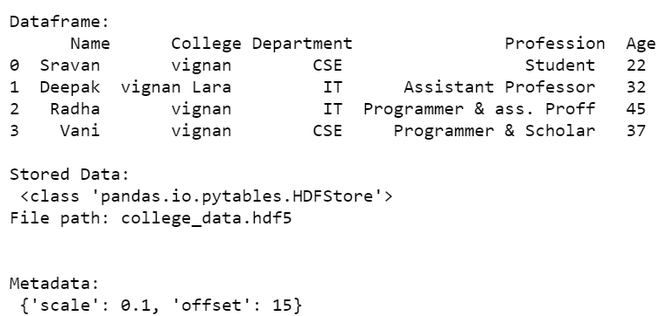Example 2

Series data structure in pandas will not support info and all methods. So we directly create metadata and display.

## Python3

 `# import required module` `import` `pandas as pd`   `# initialise data of lists using dictionary.` `data ``=` `{``'Name'``: [``'Sravan'``, ``'Deepak'``, ``'Radha'``, ``'Vani'``],` `        ``'College'``: [``'vignan'``, ``'vignan Lara'``, ``'vignan'``, ``'vignan'``],` `        ``'Department'``: [``'CSE'``, ``'IT'``, ``'IT'``, ``'CSE'``],` `        ``'Profession'``: [``'Student'``, ``'Assistant Professor'``,` `                       ``'Programmer & ass. Proff'``,` `                       ``'Programmer & Scholar'``],` `        ``'Age'``: [``22``, ``32``, ``45``, ``37``]` `        ``}`   `# Create series` `ser ``=` `pd.Series(data)`   `# display data` `ser`

Output: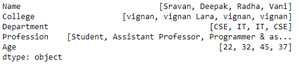Now we will store the metadata and then display it.

## Python3

 `# storing data in hdf5 file format` `storedata ``=` `pd.HDFStore(``'college_data.hdf5'``)`   `# data` `storedata.put(``'data_01'``, ser)`   `# mentioning scale and offset` `metadata ``=` `{``'scale'``: ``0.1``, ``'offset'``: ``15``}`   `storedata.get_storer(``'data_01'``).attrs.metadata ``=` `metadata`   `# storing close` `storedata.close()`   `# getting attributes` `with pd.HDFStore(``'college_data.hdf5'``) as storedata:` `    ``data ``=` `storedata[``'data_01'``]` `    ``metadata ``=` `storedata.get_storer(``'data_01'``).attrs.metadata`   `# display data` `print``(``'\nData:\n'``, data)`   `# display stored data` `print``(``'\nStored Data:\n'``, storedata)`   `# display Metadata` `print``(``'\nMetadata:\n'``, metadata)`

Output: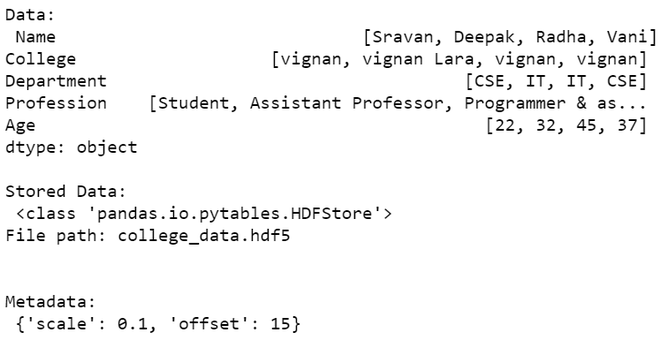Whether you're preparing for your first job interview or aiming to upskill in this ever-evolving tech landscape, GeeksforGeeks Courses are your key to success. We provide top-quality content at affordable prices, all geared towards accelerating your growth in a time-bound manner. Join the millions we've already empowered, and we're here to do the same for you. Don't miss out - check it out now!

Previous
Next